PHY294H - Lecture 34

In a series LRC combination with applied AC voltage,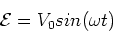(1)

The current in the circuit is given by,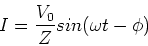(2)

The phase,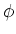is given by,(3)

and the impedance, Z, is given by,

 Z = [(XL-XC)2 + R2]1/2 (4)

where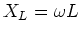and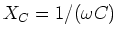.

LRC circuit demo

We have an LRC box, with L fixed at L=1Hand R, C, and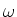variable. We set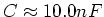and. We then have,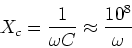(5)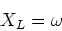(6)

We then expect that resonance will occur at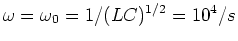. The frequency in Hertz is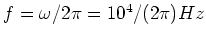For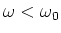, the capacitor has higher impedance than the inductor. The current in the circuit should be ahead of the voltage. This means that the phase should be negative as it is. For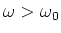, the inductive reactance is higher than the capacitive reactance, so the applied voltage leads the current in the circuit. In this case, the phase is positive. The voltage across the resistor is in phase with the current across the resistor, so we use the voltage across the resistor to illustrate the phase of the current as a function of frequency. At all frequencies, the voltage across the capacitor should lag the current by, while the voltage across the inductor leads the current by.

Power dissipated in an LRC circuit

As we noted before ideal inductors and capacitors only store and release energy, they do not dissipate energy. The energy is only dissipated in the resistor. The power dissipated is then,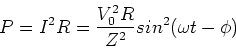(7)

if we average the sine function over a period, we get the average power (also called the rms power),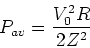(8)

The power dissipation is maximum at resonance, where Pav = V02/(2R). A plot of the power as function of frequency shows a resonant peak, as expected. This peak is higher for smaller resistance. Two measures of the width of the resonant peak are: The half width, which is the frequency width at which the resonant peak reduces to half of its peak value,. It is easy to show that,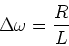(9)

The second measure is the quality factor, Q, which is defined by,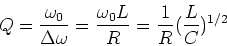(10)

Power factor

Often the power dissipated in an LRC circuit is given as,(11)

This is true as we proved last lecture that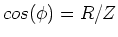. The term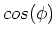is called the power factor as it gives the reduction in power as compared to one in which the entire impedance Zcontributes to the dissipation. The power factor tells us what fraction of the load is resistive.

Rectifiers and filters

Half-wave and full-wave rectifiers. AC and DC filters using capacitors and inductors.

Impedance matching

If we want to deliver maximum power from a source circuit with impedance Z1 to a load circuit with impedance Z2, it is best to use,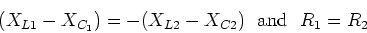(12)